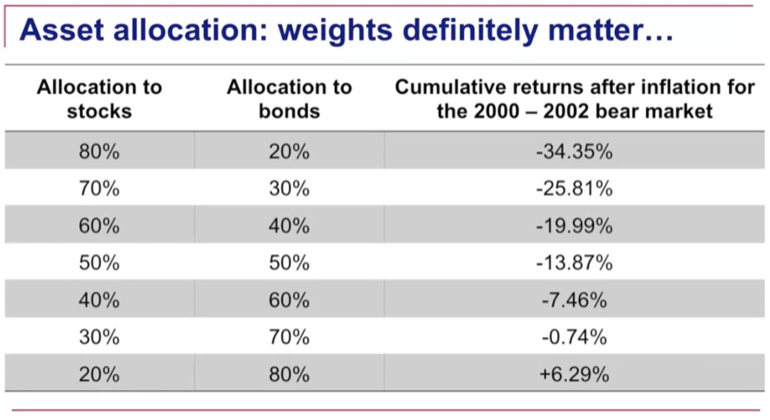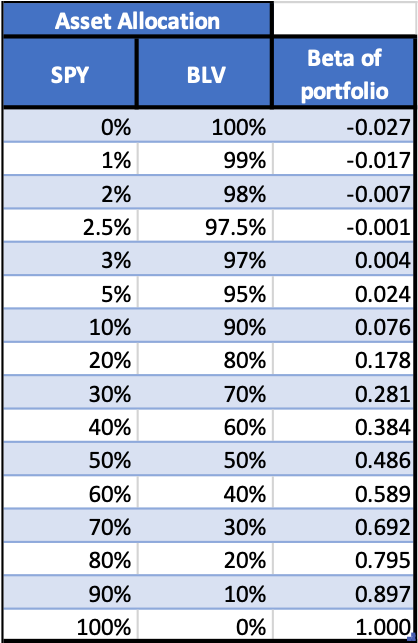# Portfolio Beta Calculator

By Arturo Barrantes
Last updated: Oct 15, 2021

This portfolio beta calculator is an outstanding tool that indicates the volatility of your investments relative to a benchmark – in this case, the stock market. This article will cover what portfolio beta is in the stock market, how to calculate the beta of a portfolio, its formula, and we conclude with a real-life example. You will never again have to wonder how to find the beta of a portfolio!

## What is the beta of a portfolio?

We start with a brief beta definition in stock market context. As mentioned in the beta calculator, the beta of a stock or the beta of a portfolio is a value that measures the extra risk we take over the market risk.

We divide risk into systematic and unsystematic risk. The first relates to the intrinsic stock market risk. It represents the risk you cannot mitigate, even by diversifying. On the other hand, the unsystematic one represents the risk of your individual asset. In other words, the additional risk you take when you invest in a particular investment. Has your company got problems in their supply chain or delivering their products? The beta of a stock intends to measure that extra risk over the market risk.

Similarly, a portfolio made by different assets will have an inherent risk over the market unless, of course, you invest in a market index like the SPY ETF. In such a case, the beta of your portfolio would be equal to the market, which is 1. Contrarily, if your portfolio differs from the assets of the market – let's say, by having fewer companies – then your portfolio beta can be smaller or bigger than 1.

We know what the beta of a portfolio is. Let's now talk mathematics to find out how to find portfolio beta.

## How to calculate the beta of a portfolio?

You first need to find the stocks' beta in your selected portfolio to start the calculation.

Such information can be obtained manually as explained in our beta-stock calculator which uses a statistical approach including covariance and variance, or through Yahoo finance. Then you have to follow the next steps:

1. First, define your asset allocation percentage. That means for 100% percent of the investable money you have, you would have to define how much you are going to invest in each asset.

We recommend you check the revenue growth and the interest coverage ratio of your desired assets, as well as their market capitalization in order to properly decide their concentration in your portfolio.

2. Second, gather the beta of each stock accordingly to the beginning paragraph of this section. Here you can take a step further by using their beta in the CAPM model to calculate an expected return.

3. Finally, we can use our fantastic portfolio beta calculator or calculate by hand by using the following formula:

βportfolio = βasset 1 * ωasset 1 + βasset 2 * ωasset 2 + ... + βasset n * ωasset n

where βasset i represents the beta of the i-th stock and ωasset i represents the asset allocation percentage (concentration) of this stock.

As you can see, it's just a weighted average formula. We will explore the importance of the portfolio beta in the next section. Keep reading.

## A portfolio beta calculation example

Lets consider the following assets and their allocations: Fortinet (β = 1.12; ω = 50%), Johnson & Johnson (β = 0.7; ω = 25%), and the market, SPY (β = 1; ω = 25%).

Then, we use the portfolio beta formula and we get:

βportfolio = 1.12 * 0.5 + 0.7 * 0.25 + 1 * 0.25 = 0.985

For the given asset allocation, your portfolio is slightly less volatile than the market (1.5% less), meaning that in a bull market, your asset will underperform the benchmark. Contrarily, in a bear market, your investment will be more defensive than the benchmark and produce lower losses.

By the way, we could have used our fast portfolio beta calculator and get the same result, just faster. Do you want to find the best asset allocation combination for this asset? If so, we recommend you check the sharpe ratio calculator.

## How to interpret the beta of a portfolio?

There can be five situations we will explain:

1. β > 1A portfolio in this category will swing more wildly than the market. With β = 1.5, if the market loses 10%, the portfolio would lose 15%. Vice-versa, the portfolio would gain 15% for a market gain of 10%. Portfolios that focus on technological and innovative companies are usually here.

2. β = 1It means the portfolio mirrors the returns of the market to which it is compared. If the benchmark goes up 10%, the portfolio will go exactly 10%. A portfolio in a mutual fund that emulates the S&P 500 would have β = 1.

3. 0 < β < 1The portfolio is less volatile than the benchmark. An example of stocks that can build a portfolio like this would be the well-positioned, anti-recession businesses like Coca-Cola or Johnson & Johnson. Such companies will make your portfolio experience less percentual drawdown compared to the market because they will anchor your portfolio with their reduced volatility.

4. β = 0 — The portfolio shows no correlation with the market. A portfolio full of cash, for example, is considered to have zero correlation with the returns of the market. It will keep its value (considering no inflation) regardless if the broad market goes up or down.

5. β < 0 — The portfolio has an opposite correlation compared to the benchmark. It means when the market goes up; the portfolio goes down and vice-versa. The Vanguard Long-Term Bond Index ETF (BLV) showed a negative beta compared to the SPY ETF from 2011 to 2019, meaning that a highly concentrated portfolio in the BLV would result in a negative beta.

## What is the importance of the portfolio beta?

Check the following picture:The following ETFs can represent the assets mentioned in the chart: SPY (an ETF that tracks the S&P 500) will represent stocks, and the Vanguard Long-Term Bond Index ETF (BLV) will represent bonds. They have a beta of 1 and -0.027, calculated with our stock beta calculator and using daily prices from the last five years.

Now, let's run a portfolio beta calculation example for different asset allocation:Note that we get a wide range of allocation possibilities for our beta. For a SPY allocation of 2.5% and the rest of the portfolio into BLV, we would bring positive returns in case of a market crash because of negative portfolio beta.

As a final comment, we can reduce our unsystematic risk by acquiring the asset at a discounted price. That can be obtained through the discounted cash flow model.
Furthermore, we invite you to check all our financial calculators to get a broad understanding of investing.

## FAQ

### Why should I care about the beta of my portfolio?

The beta of a portfolio indicates how much extra volatility your portfolio has compared to the market. Volatility is the representation of the risk of your current investments. Thus, the more volatility (higher beta) indicates that your portfolio will swing more wildly than the market and book a loss in case of panic sell. Consequently, we design asset allocation to produce portfolio beta with a risk that the investor can bear.

### What is a good beta for a portfolio?

A beta for a portfolio is as good as the risk the investor can bear. The bigger the beta, the greater the risk of a drawdown. Also, the greater the possibility of obtaining better results than the market. The opposite is the same with a lower beta (fewer returns but fewer drawdowns). Investors have to define which beta is appropriate for them.

### What is the beta of the all-weather portfolio of Ray Dalio?

Based on the last 2 year of data prices (10-2019 to 10-2021) and considering the following ETF, their betas and their asset allocation percentage are GSG (β = 0.53; ω = 7.5%), GLD (β = 0.06; ω = 7.5%), IEI (β = -0.06; ω = 15%), TLT (β = -0.32; ω = 40%), and VTI (β = 1.02; ω = 30%). Now we input this information into Omni's portfolio beta calculator and see that the portfolio β equals 0.213, meaning the portfolio is 78.68% less volatile than the market. A good choice for market crashes!

### How do I calculate the beta of a portfolio?

Here are the steps to follow when you want to compute portfolio beta:

1. You need the beta of the stocks. You can calculate them yourself by gathering the daily stock returns from at least the last two years or using Omni's beta stock calculator.
2. You have to decide on the asset allocation percentage. This step is critical because it will directly influence the beta of the portfolio calculation.
3. Compute the weighted average of the asset betas with weights given by their respective allocation percentages. The result is the beta of your portfolio. You can also try Omni's excellent beta portfolio calculator.
Arturo Barrantes
Stock weight in your portfolio and it's respective beta
1st stock weight
%
1st stock beta
2nd stock weight
%
2nd stock beta
Enter at least 2 assets percentage allocation and their respective beta.
People also viewed…

### Appreciation

The appreciation calculator is a tool that helps you find the future value of anything based on a provided appreciation rate, or find appreciation rate based on future value.

### Days inventory outstanding (DIO)

Our DIO calculator (days inventory outstanding) allows you to calculate the time it takes for a company to turn its inventory into sales.

### Helium balloons

Wondering how many helium balloons it would take to lift you up in the air? Try this helium balloons calculator! 🎈